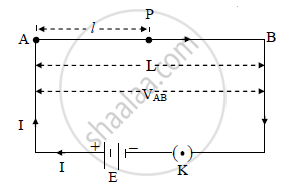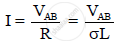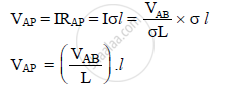HSC Science (Electronics) 12th Board ExamMaharashtra State Board
Share

# Explain the Principle of Potentiometer. - HSC Science (Electronics) 12th Board Exam - Physics

#### Question

Explain the principle of potentiometer.

#### Solution

Principle:

The potential difference between any two points of the potentiometer wire is directly proportional to the length of wire between these two points.

The fall of potential per unit length of potentiometer wire (potential gradient of wire) is
constant.

Explanation:

a) Suppose a potentiometer wire AB of length L and resistance R is stretched on the
rectangular wooden board. The source of e.m.f E and negligible internal resistance is
connected to the wire AB through a key K as shown in figure.b) Resistance per unit length of wire AB is given by, σ = R/L

∴ R = σL

c) Let ‘VAB’ be the P.D across the wire. There is uniform fall of potential along the wire
from A to B.

d) By Ohm’s law, the current ‘I’ passing through the wire is given by,e) Let ‘P’ be any point on the wire, such that AP = ‘l’. The resistance of wire AP of
length ‘l’ is RAP = σl. So the potential difference ‘V’AP between points A and P is given
by,f) But, VAB and L are constant,

∴ VAP ∝ L

∴ V_(AP)/L = K = constant

where, K is called potential gradient of wire.

Is there an error in this question or solution?

#### APPEARS IN

2012-2013 (October) (with solutions)
Question 7.1 | 2.00 marks
Solution Explain the Principle of Potentiometer. Concept: Meter Bridge.
S